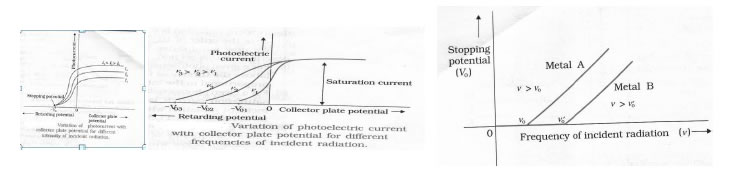Chapter 7 Dual Nature Of Matter & Radiation

• The elementary particle in an atom having negative charge of magnitude 1.6 x 10-19 C and mass of 9.1 x 10-19 kg is called electron.

• There are four types of electron emission, namely, Thermionic Emission, Photoelectric Emission, Field Emission and secondary emission.

• The minimum energy required by an electron to escape from the metal surface is called work function.

• Work function is conveniently expressed in electron volts ( e V ).

• One electron volt is the energy gained or lost by an electron while passing through a potential difference of one volt.

• The phenomenon of emission of electrons from metal surface when suitable high frequency radiation incidents on it is called photoelectric effect. The electrons thus emitted are called photo electrons.

• The electric current in the circuit constituting the photo electrons is called photoelectric current. It depends on the intensity of incident radiation, potential difference applied between the two electrodes and the nature of the material.

• The minimum negative voltage required to stop completely the photo electric current is called stopping potential.

• The frequency of incident radiation below which no photo electric effect takes place is called threshold frequency.

#### • Laws of photoelectric effec t:-

1. The photoelectric emission is an instantaneous process.
2. For a given metal and frequency of radiation the photoelectric current is directly proportional to the intensity of incident radiation.
3. For a given metal if the frequency of incident radiation below its threshold frequency then no photoelectric effect takes place.
4. The maximum kinetic energy of photo electron is directly proportional to the frequency of incident radiation and independent of its intensity.

### EXPERIMENTAL STUDY OF PHOTOELECTRIC EFFECT##### The stopping potential Vo depends on

i) The frequency of incident light and
ii) the nature of emitter material. For a given frequency of incident light, the stopping potential is independent of its intensity. eVo =(1/2)m v 2 max =kmax

• The light propagates in the form of wave and interacts with matter in the form of discrete packets of energy called quantum of energy. One quantum of light radiation is called PHOTON.

• A photon travels with the speed of light

• Frequency of photon does not change as it travels from one medium to another but wavelength changes as speed changes.

• The rest mass of photon is zero as the photon can not exist in rest.

• Energy of photon E = hν = hc/λ

• Momemntum of photon is p = mc = hν/c = h/λ

• The equivalent mass of photon is m = E/c2 = hν/c2

• Einstein’s photo electric equation:

Kmax = hν – φ0 or eV0 = hν - φ0 where φ0is the work function of metal.

##### • Radiation has dual nature :-

wave and particle. The wave nature is revealed in phenomenon like interference, diffraction and polarization. The particle nature is revealed by the phenomenon photo electric effect.

• By symmetry, matter also should have dual nature: wave and particle. The waves associated with the moving material particle are called matter waves or De Broglie waves.

• The De Broglie wave length (λ) associated with the moving particle is related to its moment p as: λ =h/p = h/mv.

• An equation for the De Broglie wavelength of an electron accelerated through a potential V.

• Consider an electron with mass ‘m’ and charge ‘e’ accelerated from rest through a potential V.
K = eV
K = 1/2mv2 = p2/2m
P2 = 2mK
P = √2mK = √2meV
λ = h/ √2meV
Substituting numerical values of h, m and e
λ = (1.227/√### NCERT Books Free Pdf Download for Class 5, 6, 7, 8, 9, 10 , 11, 12 Hindi and English Medium

 Mathematics Biology Psychology Chemistry English Economics Sociology Hindi Business Studies Geography Science Political Science Statistics Physics Accountancy